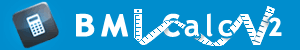# Harris-Benedict Equation

Harris-Benedict Equation calculates the Basal Metabolic Rate and helps determine daily calorie requirements. Harris-Benedict Equation was proposed by J. A. Harris and F. G. Benedict in the book "A Biometric Study of Basal Metabolism in Man" published in 1919.

To determine daily calorie requirements, the value of Basal Metabolic Rate is multiplied by a factor that reflects the activity level of an individual. The result is the recommended daily calorie intake to maintain existing body weight. It is important for athletes who are building muscle mass. If you are trying to lose or gain weight, it is very helpful to know how many calories you burn in a day. Harris-Benedict Equation is a mathematical approach to weight loss. Harris-Benedict Equation may help in weight loss showing the way to reducing the calorie intake below the calculated maintenance intake.

To use Harris Benedict Equation, you must determine the Basal Metabolic Rate which is the number of calories burned in a day at complete rest without any additional activity. The Basal Metabolic Rate depends on sex, age and body size. However, Basal Metabolic Rate takes into account only the average ratio of lean muscle mass to body weight. Harris Benedict Equation may be incorrect for very muscular persons, underestimating their real needs, and for very fat people, overestimating their real needs. Basal Metabolic Rate from Harris-Benedict Equation expressed in calories serves as a baseline. Then, the value of Basal Metabolic Rate is multiplied by a factor that depends on individual daily activity to get daily calorie requirements.

Harris and Benedict showed that the values could be computed from height, weight, age, and sex. You can see practical application of Harris-Benedict Equation calculating your BMR with BMR calculator for women or BMR calculator for men.

## Calculating the BMR

### Early Harris-Benedict equations published in 1918 and 1919

Men: BMR = 66.4730 + (13.7516 x weight in kg) + (5.0033 x height in cm) – (6.7550 x age in years)

Women: BMR = 655.0955 + (9.5634 x weight in kg) + (1.8496 x height in cm) – (4.6756 x age in years)

### Harris-Benedict equations revised by Roza and Shizgal

Harris-Benedict Equation was reevaluated from the standpoint of resting energy requirements and the body cell mass. In 1984, Roza and Shizgal showed that resting energy expenditure is directly related to the size of body cell mass and is independent of age and sex. The variables in Harris-Benedict Equation reflect the relationship between body weight and the body cell mass.

Men: BMR = 88.362 + (13.397 x weight in kg) + (4.799 x height in cm) - (5.677 x age in years)

Women: BMR = 447.593 + (9.247 x weight in kg) + (3.098 x height in cm) - (4.330 x age in years)

## Application of Harris-Benedict Equation

### Calculation of recommended daily calorie intake to maintain present weight

Little activity (no exercise): Daily kilocalories recommended = BMR x 1.2

Light activity (exercise 1–3 days per week): Daily kilocalories recommended = BMR x 1.375

Moderate activity (exercise 3–5 days per week): Daily kilocalories recommended = BMR x 1.55

Heavy activity (exercise 6–7 days per week): Daily kilocalories recommended = BMR x 1.725

Very heavy activity (extra heavy workouts): Daily kilocalories recommended = BMR x 1.9

* Pregnancy: Daily kilocalories recommended = Daily kilocalories needed + 300 kilocalories

** Lactation: Daily kilocalories recommended = Daily kilocalories needed + 500 kilocalories